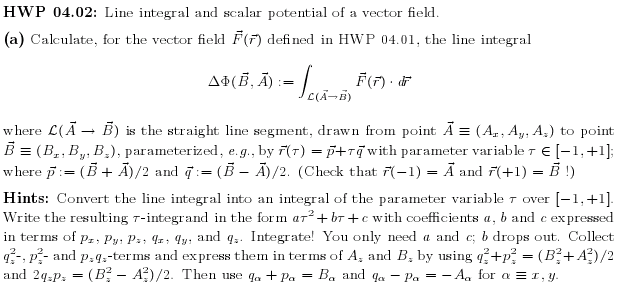# Scalar potential and line integral of a vector field

alecst

## Homework StatementGiven above.

## The Attempt at a Solution

I attempted this problem first without looking at the hint.

I've defined F(r) as (B+A)/2 + t(B-A)/2, with dr as (B-A)/2 dt . Thus F(r)dr = ((B+A)/2)*((B-A)/2)+((B-A)/2)^2 dt

When I integrate this from -1 to 1 I get 1/2*(B^2-A^2).

When I then looked at the hint, I saw it mentioned another (B^2+A^2)/2 term and another "c," neither of which I have, and my integrand has no "tau" squared element either. Is there a point where I went wrong here?